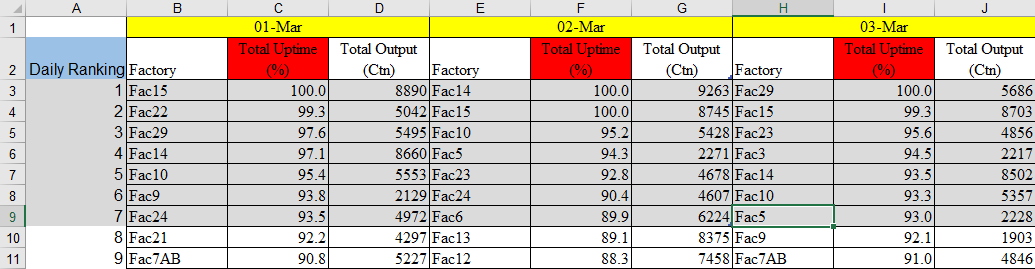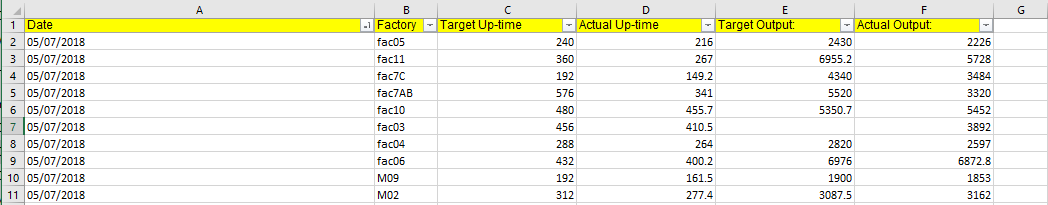# Qlik Sense App Development

Discussion board where members can learn more about Qlik Sense App Development and Usage.

New Contributor III

## Ranking in Pivot Table (Qlik Sense)

Hello everyone,

I have a problem where I have to make a pivot table that looks exactly like this .In the raw file, The data looks like thisRequirement :

1) Ranking is calculated based on the total uptime

2) The 1st priority is the output should be as the first image

Calculation :

Total uptime % = (Actual uptime / target uptime) * 100

Total Output = (Actual output/ target output) * 100

Rank (sum((Actual uptime / target uptime) ))

My problem right now :

1) Daily ranking is not exist and unavailable to be put as dimension

2) Factory should logically be the dimension in the pivot table

3) Pivot table in qlik sense cannot be sorted by measure

My current solution :I ranked it using the rank formula. I have not found any other solution so far.

Can someone help me solve this problem? or is it impossible?

Thank you in advance!

Tags (1)